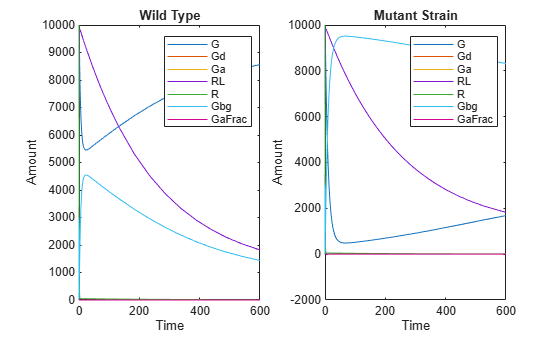## Simulate Biological Variability of the Yeast G Protein Cycle Using Wild-Type and Mutant Strains

This example shows how to create and apply a variant to the G protein model of a wild-type strain. The variant represents a parameter value for the G protein model of a mutant strain. Thus, when you simulate the model without applying the variant, you see results for the wild type strain, and when you simulate the model with the variant, you see results for the mutant strain. This example uses the model described in Model of the Yeast Heterotrimeric G Protein Cycle.

The value of the parameter `kGd` is `0.11` for the wild-type strain and `0.004` for the mutant strain. To represent the mutant strain, you will store an alternate value of `0.004` for the `kGd` parameter in a variant object, and apply this variant when simulating the model.

For information on variants, see Variants in SimBiology Models.

Load the `gprotein.sbproj` project, which includes the variable `m1`, a SimBiology® model object.

`sbioloadproject gprotein`

You can create a variant of the original model by specifying a different parameter value for the `kGd` parameter of the model. First, add a variant to the `m1` model object.

`v1 = addvariant(m1,'mutant_strain');`

Next, add a parameter `kGd` with a value of `0.004` to the variant object `v1`.

`addcontent(v1,{'parameter','kGd','Value',0.004});`

Simulate the wild type model.

`[t,x,names] = sbiosimulate(m1);`

Simulate the mutant strain model by applying the variant.

`[tV,xV,names] = sbiosimulate(m1,v1);`

Plot and compare the simulated results.

```subplot(1,2,1) plot(t,x); legend(names); xlabel('Time'); ylabel('Amount'); title('Wild Type'); subplot(1,2,2) plot(tV,xV); legend(names); xlabel('Time'); ylabel('Amount'); title('Mutant Strain');```